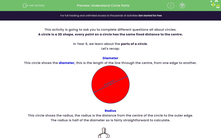# Understand Circle Parts

In this worksheet, students will be asked to find parts of a circle, the diameter, radius and circumference.Key stage:  KS 2

Curriculum topic:   Geometry: Properties of Shapes

Curriculum subtopic:   Know Circle Parts

Popular topics:   Geometry worksheets

Difficulty level:#### Worksheet Overview

This activity is going to ask you to complete different questions all about circles.

A circle is a 2D shape, every point on a circle has the same fixed distance to the centre.

In Year 6, we learn about the parts of a circle.

Let's recap:

Diameter

This circle shows the diameter, this is the length of the line through the centre, from one edge to another.This circle shows the radius, the radius is the distance from the centre of the circle to the outer edge.

The radius is half of the diameter so is fairly straightforward to calculate.Circumference

A circle's circumference is the distance around the edge.How to find the circumference of a circle

To find the circumference of a circle we have to use the formulae:

(2 xx r)= pi = 3.14

Example:So, let's find the circumference of this circle.

(2 x 3.14 x 5) =

(6.28 x 5) = 31.4

The circumference is 31.4cm